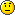SB Physics Help Room Sucks!
Would you like to react to this message? Create an account in a few clicks or log in to continue.

# 23.3 Maple TA##23.3 Maple TA

QUESTION 1

QUESTION 2

QUESTION 3

QUESTION 4

Wavelength given *[SQRT[(1+(%GIVEN /100)) / (1-(% GIVEN/100))]]=answer

PHYZ
Guest

##Question #2

PHYZ...for some reason number two is not working for me..this is what i did...(1/(sqrt(1-(0.1234^2)))^-1= -0.9924...and its not working ..pls help???

Guest001
Guest

##Re: 23.3 Maple TA

It's not to the negative power, its the fraction and then minus 1.

So you should get 1/sqrt(.98477) = 1.0077 -1 = .0077 is your answer. and then negative of course

Kathleen

Posts : 42
Join date : 2008-12-18

##question #2

thanks alot kathleen!!!

guest001
Guest

##Re: 23.3 Maple TA

I'm not able to get an answer for #4-- and I agree with your equation (according to the slides...) Is anyone else having this problem?

Thanks,

S
Guest

##Re: 23.3 Maple TA

S wrote:I'm not able to get an answer for #4-- and I agree with your equation (according to the slides...) Is anyone else having this problem?

Thanks,

The provided equation is correct.

Are you:

1. Making sure to convert the percentage into decimal form? (i.e. 9.57% = .0957)
2. Watching your signs? (the fraction under the square root has 1+ as the numerator, and 1- as the denominator)

DJ

Posts : 28
Join date : 2009-03-17

##Re: 23.3 Maple TA

Thanks so much! Actually I made the stupid mistake of not converting my percents properly...

S
Guest

##Re: 23.3 Maple TA

4) A galaxy receding from us with 11.484 % of the speed of light emits light at a wavelength of 525.9 nm. What is the wavelength we mesure in nm (sheet 35) ? Indicate with a negative (positive) sign whether the result changes (is the same) for the galaxy approaching us.
(525.9 E-9)* sqrt[ (1 + (11.484 / 100) / (1- (11.484/ 100)) =
525E-9 * sqrt (1.11484/ 0.88516) =
525E-9 * 1.122265= 5.8919E-7

I keep getting this answer and i think its right but it keeps coming out wrong. Can anyone help me with this one? I will greatly appreciate it!!!Money23
Guest

##Re: 23.3 Maple TA

money,

4) A galaxy receding from us with 11.484 % of the speed of light emits light at a wavelength of 525.9 nm. What is the wavelength we mesure in nm (sheet 35) ? Indicate with a negative (positive) sign whether the result changes (is the same) for the galaxy approaching us.
(525.9 E-9)* sqrt[ (1 + (11.484 / 100) / (1- (11.484/ 100)) =
525E-9 * sqrt (1.11484/ 0.88516) =
525E-9 * 1.122265= 5.8919E-7

I keep getting this answer and i think its right but it keeps coming out wrong. Can anyone help me with this one? I will greatly appreciate it!!!

don't convert wavelength into SI, leave in nm. You're answer: -590.199

hax0r
Guest

THANK YOU!!!

Money23
Guest

##Re: 23.3 Maple TA